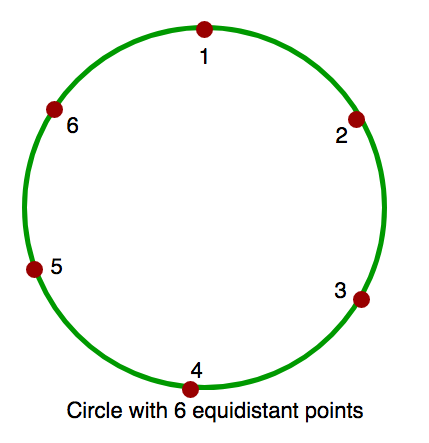# Count of obtuse angles in a circle with ‘k’ equidistant points between 2 given points

• Difficulty Level : Medium
• Last Updated : 22 Jun, 2022

A circle is given with k equidistant points on its circumference. 2 points A and B are given in the circle. Find the count of all obtuse angles (angles larger than 90 degree) formed from /_ACB, where C can be any point in circle other than A or B.
Note :
A and B are not equal.
A < B.
Points are between 1 and K(both inclusive).Examples :

```Input : K = 6, A = 1, B = 3.
Output : 1
Explanation : In the circle with 6
equidistant points, when C = 2 i.e.
/_123, we get obtuse angle.

Input : K = 6, A = 1, B = 4.
Output : 0
Explanation : In this circle, there
is no such C that form an obtuse angle.```

It can be observed that if A and B have equal elements in between them, there can’t be any C such that ACB is obtuse. Also, the number of possible obtuse angles are the smaller arc between A and B.
Below is the implementation :

## C++

 `// C++ program to count number of obtuse``// angles for given two points.``#include ``using` `namespace` `std;` `int` `countObtuseAngles(``int` `a, ``int` `b, ``int` `k)``{``    ``// There are two arcs connecting a``    ``// and b. Let us count points on``    ``// both arcs.``    ``int` `c1 = (b - a) - 1;``    ``int` `c2 = (k - b) + (a - 1);` `    ``// Both arcs have same number of``    ``// points``    ``if` `(c1 == c2)``        ``return` `0;` `    ``// Points on smaller arc is answer``    ``return` `min(c1, c2);``}` `// Driver code``int` `main()``{``    ``int` `k = 6, a = 1, b = 3;``    ``cout << countObtuseAngles(a, b, k);``    ``return` `0;``}`

## Java

 `// Java program to count number of obtuse``// angles for given two points``class` `GFG {` `    ``static` `int` `countObtuseAngles(``int` `a,``                                 ``int` `b, ``int` `k)``    ``{` `        ``// There are two arcs connecting a``        ``// and b. Let us count points on``        ``// both arcs.``        ``int` `c1 = (b - a) - ``1``;``        ``int` `c2 = (k - b) + (a - ``1``);` `        ``// Both arcs have same number of``        ``// points``        ``if` `(c1 == c2)``            ``return` `0``;` `        ``// Points on smaller arc is answer``        ``return` `min(c1, c2);``    ``}` `    ``// Driver Program to test above function``    ``public` `static` `void` `main(String arg[])``    ``{` `        ``int` `k = ``6``, a = ``1``, b = ``3``;``        ``System.out.print(countObtuseAngles(a, b, k));``    ``}``}` `// This code is contributed by Anant Agarwal.`

## Python

 `# C++ program to count number of obtuse``# angles for given two points.` `def` `countObtuseAngles( a, b, k):``    ``# There are two arcs connecting a``    ``# and b. Let us count points on``    ``# both arcs.``    ``c1 ``=` `(b ``-` `a) ``-` `1``    ``c2 ``=` `(k ``-` `b) ``+` `(a ``-` `1``)`` ` `    ``# Both arcs have same number of``    ``# points``    ``if` `(c1 ``=``=` `c2):``       ``return` `0``     ` `    ``# Points on smaller arc is answer``    ``return` `min``(c1, c2)`` ` `# Driver code``k, a, b ``=` `6``, ``1``, ``3``print` `countObtuseAngles(a, b, k)` `# This code is contributed by Sachin Bisht`

## C#

 `// C# program to count number of obtuse``// angles for given two points``using` `System;` `class` `GFG {` `    ``static` `int` `countObtuseAngles(``int` `a,``                           ``int` `b, ``int` `k)``    ``{` `        ``// There are two arcs connecting``        ``// a and b. Let us count points``        ``// on both arcs.``        ``int` `c1 = (b - a) - 1;``        ``int` `c2 = (k - b) + (a - 1);` `        ``// Both arcs have same number``        ``// of points``        ``if` `(c1 == c2)``            ``return` `0;` `        ``// Points on smaller arc is``        ``// answer``        ``return` `Math.Min(c1, c2);``    ``}` `    ``// Driver Program to test above``    ``// function``    ``public` `static` `void` `Main()``    ``{` `        ``int` `k = 6, a = 1, b = 3;``        ` `        ``Console.WriteLine(``           ``countObtuseAngles(a, b, k));``    ``}``}` `// This code is contributed by vt_m.`

## PHP

 ``

## Javascript

 ``

Output :

`1`

Time Complexity: O(1)
Auxiliary Space: O(1)

This article is contributed by Aarti_Rathi and Rohit Thapliyal. If you like GeeksforGeeks and would like to contribute, you can also write an article using write.geeksforgeeks.org or mail your article to review-team@geeksforgeeks.org. See your article appearing on the GeeksforGeeks main page and help other Geeks.
Please write comments if you find anything incorrect, or you want to share more information about the topic discussed above.

My Personal Notes arrow_drop_up